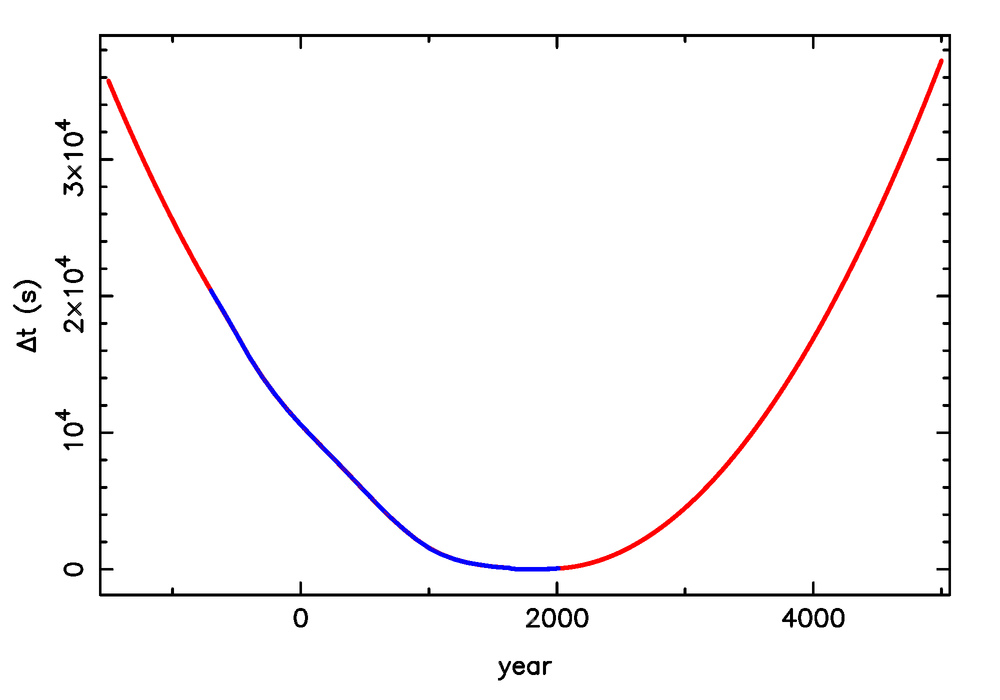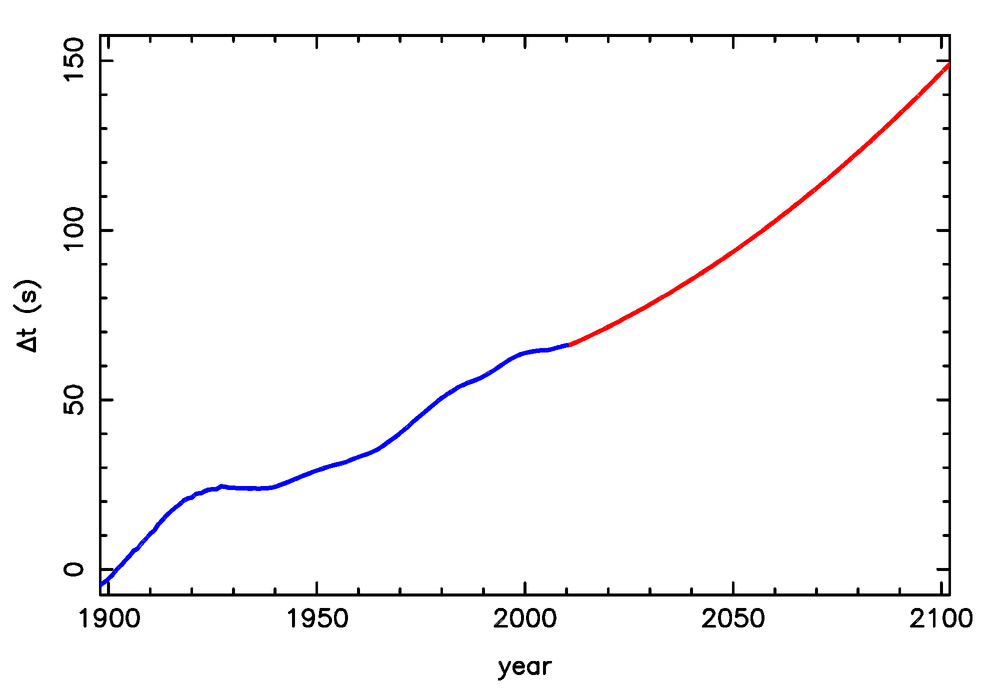Computing the heavens   -   De sterrenhemel voor Nederland en België# Extrapolation of Δt

The rotation of the Earth is not constant, but slows down over time, mainly due to the effect of tides from the Moon. The difference between a hypothetical uniformly rotating Earth and the true rotation of the Earth is expressed as the quantity Δt, which is the difference between the Universal Time (UT) and Dynamical Time (DT). However, the deceleration of the Earth is not constant, and because of that, we can only infer Δt from observations — we cannot predict it. Similarly, we do not know Δt before the year -700, from which time on historical data are available.

Obtaining a reliable value for Δt in the historical past is simple — I use a table of inferred values and a simple interpolation scheme. While for most events described on this website the exact value of Δt is unimportant, known, or both, solar eclipses in the (distant) past or future are affected more dramatically, and it would be useful to have a method to extrapolate from available data in a reasonable way. Based on a list of historical values for Δt between the years -700 and 2000, and a bit of experimentation, I have decided to use the following scheme:
1. I use a parabolic prescription, expressing Δt in seconds and t in years:
Δt(t)  =  Δt0  +  dΔt/dt × (t-t0)  +  d2Δt/dt2 × (t-t0)2
2. I use the last known value of Δt, called Δt0, at each end of the interval, measured at time t0. For t0=-700, Δt0=2.04×104 s, for t0=2010, Δt0=66.0699 s.
3. For dΔt/dt, I take the mean change in the 100 years closest to t0. In -700 this amounts to -16 s/yr, in 2010 this results in 0.551 s/yr (55.1 s/century).
4. I did a parabolic fit on the historical data between the years -700 and 2000, and use the quadratic coefficient of that fit for d2Δt/dt2. I find the value (3.9755±0.0048)×10-3 s/yr2 (39.755±0.048 s/century2, corresponding to a mean increase of the day length of about 2.177 ms/century), which I use for extrapolation into both the past and the future.
I think that this scheme is one of the more reasonable among the many that can be made up to predict the unpredictable. It ensures that there is no jump around t0 because of (2), that the behaviour in the near past/future is similar to that of the next/last century because of (3) and that the long-term behaviour is as desired because of (4). In addition, this behaviour allows stable adaptation when newly measured values are added to my historical list. The results of the extrapolation are shown in the figures below for the long, intermediate and short time scale. The blue line represents the historical data, the red line shows the extrapolation. Click on an image to see it in its full size.I used the following (historical) data:
A machine-readable table of these compiled data, up to 2019, is deltat.dat. Predictions for Δt in the near future can be found on the IERS website as well, but the rate of change is often discontinuous between e.g. the first and later years. My extrapolated values for the near and farther future (as of 2011), obtained using the method described above, can be found in DeltaT_pred.dat. Please use it with a pack of salt.

## Definitions

• Δt = 32.184s + (TAI-UTC) - (UT1-UTC) = 32.184s + TAI - UT1 = TT - UT1. Cumulative difference between “idealised time in the universe” or “dynamical time” and “clock time”. Δt was negative between ∼ 1871 and 1902, and positive at all other times (barring giant impacts).
• TAI-UTC = cumulative number of leap seconds = 35s on 2012-07-01; changes every now and then by one second.
• UT1-UTC = ∼ -0.6 – +0.4 s; measured by e.g. the IERS.
• TAI-GPS = 19s, and fixed.
• GPS-UTC = (TAI-UTC) - (TAI-GPS) = 35s - 19s = 16s on 2012-07-01; changes when a leap second is introduced.

• TAI = Temps Atomique International; atomic time based on continuous counting of the SI second; independent of the rotation of the Earth. Approximation of TT, but affected by relativistic effects.
• UTC = Coordinated Universal Time; “clock time”, differs from local time by the time zone (ususally an (semi)integer number of hours), updated only by leap seconds to stay in touch with the Earth's rotation.
• UT1 = Universal Time 1; changes continuously, since the rotation of the Earth is variable. When UT1-UTC reaches ∼-0.6s, a leap second is introduced, which changes UTC such that UT1-UTC ∼+0.4s.
• TT = Terrestrial Time; replaces “Terrestrial Dynamical Time” (TDT) and “Ephemeris Time” (ET); TT ≈ TAI + 32.184s ≈ GPS + 51.184s, independent of the Earth's rotation. Idealised concept, approximated by TAI, but without relativistic effects.

Computing the heavens   -   De sterrenhemel voor Nederland en België